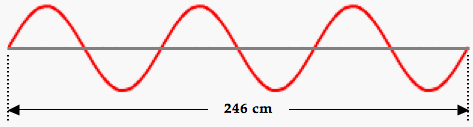# Waves, Sound and Light: Wave Basics

## Wave Basics: Audio Guided Solution

#### Problem 24:

A standing wave pattern is established in a 246-cm long rope. A snapshot of the rope at a given moment in time is shown in the diagram below. Vibrations travel within the rope at speeds of 22.7 m/s. Determine the frequency of vibration of the rope.## Habits of an Effective Problem Solver

• Read the problem carefully and develop a mental picture of the physical situation. If necessary, sketch a simple diagram of the physical situation to help you visualize it.
• Identify the known and unknown quantities and record in an organized manner, often times they can be recorded on the diagram itself. Equate given values to the symbols used to represent the corresponding quantity (e.g., v = 12.8 m/s, λ = 4.52 m, f = ???).
• Use physics formulas and conceptual reasoning to plot a strategy for solving for the unknown quantity.
• Identify the appropriate formula(s) to use.
• Perform substitutions and algebraic manipulations in order to solve for the unknown quantity.